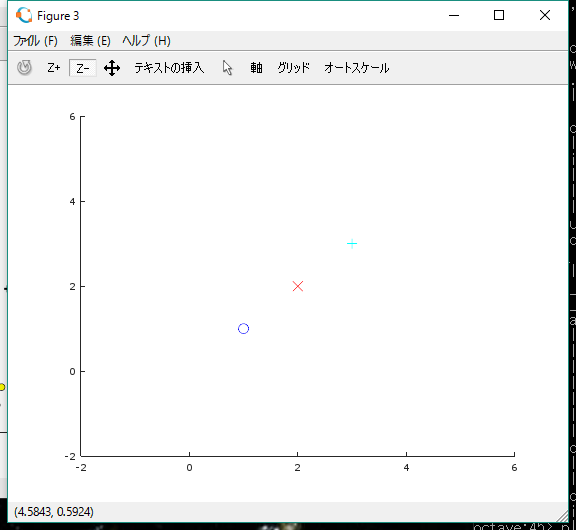# Octave Plot

```>> figure;  % 図を表示するためのウィンドウを開く
>> hold on; % 続けて表示するようにする。
>> plot(1,1,'bo', 'MarkerSize', 10);
>> plot(2,2,'rx', 'MarkerSize', 10);
>> plot(3,3,'c+', 'MarkerSize', 10);
>> hold off; %解除``````> help plot
'plot' is a function from the file C:\Octave\Octave-4.2.0\share\octave\4.2.0\m\plot\draw\plot.m

-- plot (Y)
-- plot (X, Y)
-- plot (X, Y, FMT)
-- plot (..., PROPERTY, VALUE, ...)
-- plot (X1, Y1, ..., XN, YN)
-- plot (HAX, ...)
-- H = plot (...)
Produce 2-D plots.

Many different combinations of arguments are possible.  The
simplest form is

plot (Y)

where the argument is taken as the set of Y coordinates and the X
coordinates are taken to be the range '1:numel (Y)'.

If more than one argument is given, they are interpreted as

plot (Y, PROPERTY, VALUE, ...)

or

plot (X, Y, PROPERTY, VALUE, ...)

or

plot (X, Y, FMT, ...)

and so on.  Any number of argument sets may appear.  The X and Y
values are interpreted as follows:

* If a single data argument is supplied, it is taken as the set
of Y coordinates and the X coordinates are taken to be the
indices of the elements, starting with 1.

* If X and Y are scalars, a single point is plotted.

* 'squeeze()' is applied to arguments with more than two
dimensions, but no more than two singleton dimensions.

* If both arguments are vectors, the elements of Y are plotted
versus the elements of X.

* If X is a vector and Y is a matrix, then the columns (or rows)
of Y are plotted versus X.  (using whichever combination
matches, with columns tried first.)

* If the X is a matrix and Y is a vector, Y is plotted versus
the columns (or rows) of X.  (using whichever combination
matches, with columns tried first.)

* If both arguments are matrices, the columns of Y are plotted
versus the columns of X.  In this case, both matrices must
have the same number of rows and columns and no attempt is
made to transpose the arguments to make the number of rows
match.
>||
Multiple property-value pairs may be specified,
but they must appear in pairs.
These arguments are applied to the line objects drawn by 'plot'.

Useful properties to modify are

"linestyle",
"linewidth",
"color",
"marker",
"markersize",
"markeredgecolor",
"markerfacecolor".  *Note Line Properties::.```
```     The FMT format argument can also be used to control the plot style.
It is a string composed of four optional parts:
"<linestyle><marker><color><;displayname;>".

When a marker is specified,
but no linestyle, only the markers are plotted.

Similarly, if a linestyle is specified,
but no marker, then only lines are drawn.

If both are specified then lines and markers will be plotted.

If no FMT and no PROPERTY/VALUE pairs are given,
then the default plot style is solid lines with no markers
and the color determined by the "colororder" property of the current axes.```
```     Format arguments:

linestyle

'-'  Use solid lines (default).
'--' Use dashed lines.
':'  Use dotted lines.
'-.' Use dash-dotted lines.

marker

'+'  crosshair
'o'  circle
'*'  star
'.'  point
'x'  cross
's'  square
'd'  diamond
'^'  upward-facing triangle
'v'  downward-facing triangle
'>'  right-facing triangle
'<'  left-facing triangle
'p'  pentagram
'h'  hexagram

color

'k'  blacK
'r'  Red
'g'  Green
'b'  Blue
'y'  Yellow
'm'  Magenta
'c'  Cyan
'w'  White

";displayname;"
Here "displayname" is the label to use for the plot legend.```
```     The FMT argument may also be used to assign legend labels.  To do
so, include the desired label between semicolons after the
formatting sequence described above, e.g., "+b;Key Title;".  Note
that the last semicolon is required and Octave will generate an
error if it is left out.

Here are some plot examples:

plot (x, y, "or", x, y2, x, y3, "m", x, y4, "+")

This command will plot 'y' with red circles, 'y2' with solid lines,
'y3' with solid magenta lines, and 'y4' with points displayed as
'+'.

plot (b, "*", "markersize", 10)

This command will plot the data in the variable 'b', with points
displayed as '*' and a marker size of 10.

t = 0:0.1:6.3;
plot (t, cos(t), "-;cos(t);", t, sin(t), "-b;sin(t);");

This will plot the cosine and sine functions and label them
accordingly in the legend.

If the first argument HAX is an axes handle, then plot into this
axis, rather than the current axes returned by 'gca'.

The optional return value H is a vector of graphics handles to the
created line objects.

To save a plot, in one of several image formats such as PostScript
or PNG, use the 'print' command.